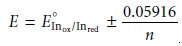Home | | Modern Analytical Chemistry | Selecting and Evaluating the End Point - Titrations Based on Redox Reactions

# Selecting and Evaluating the End Point - Titrations Based on Redox Reactions

Where Is the Equivalence Point? In discussing acidâ€“base titrations and com- plexometric titrations, we noted that the equivalence point is almost identical with the inflection point located in the sharply rising part of the titration curve.

Selecting and Evaluating the End Point

The equivalence point of a redox titration occurs when stoichiometrically equiva- lent amounts of analyte and titrant react. As with other titrations, any difference be- tween the equivalence point and the end point is a determinate source of error.

## WhereIs the Equivalence Point?

In discussing acidâ€“base titrations and com- plexometric titrations, we noted that the equivalence point is almost identical with the inflection point located in the sharply rising part of the titration curve. If you look back at Figures 9.8 and 9.28, you will see that for acidâ€“base and com- plexometric titrations the inflection point is also in the middle of the titration curveâ€™s sharp rise (we call this a symmetrical equivalence point). This makes it relatively easy to find the equivalence point when you sketch these titration curves. When the stoichiometry of a redox titration is symmetrical (one mole analyte per mole of titrant), then the equivalence point also is symmetrical. If the stoichiometry is not symmetrical, then the equivalence point will lie closer to the top or bottom of the titration curveâ€™s sharp rise. In this case the equivalence point is said to be asymmetrical. Example 9.12 shows how to calculate the equiv- alence point potential in this situation.## Finding the End Point with a Visual Indicator

Three types of visual indicators are used to signal the end point in a redox titration. A few titrants, such as MnO4â€“, have oxidized and reduced forms whose colors in solution are signifi- cantly different. Solutions of MnO4â€“ are intensely purple. In acidic solutions, however, permanganateâ€™s reduced form, Mn2+, is nearly colorless. When MnO4â€“ is used as an oxidizing titrant, the solution remains colorless until the first drop of excess MnO4â€“ is added. The first permanent tinge of purple signals the end point.

A few substances indicate the presence of a specific oxidized or reduced species. Starch, for example, forms a dark blue complex with I3â€“ and can be used to signal the presence of excess I3â€“ (color change: colorless to blue), or the completion of a reaction in which I3â€“ is consumed (color change: blue to colorless). Another exam- ple of a specific indicator is thiocyanate, which forms a soluble red-colored com- plex, Fe(SCN)2+, with Fe3+.

The most important class of redox indicators, however, are substances that do not participate in the redox titration, but whose oxidized and reduced forms differ in color. When added to a solution containing the analyte, the indicator im- parts a color that depends on the solutionâ€™s electrochemical potential. Since the indicator changes color in response to the electrochemical potential, and not to the presence or absence of a specific species, these compounds are called general redox indicators.

The relationship between a redox indicatorâ€™s change in color and the solutionâ€™s electrochemical potential is easily derived by considering the half-reaction for the indicator

Inox + neâ€“ < == == >  Inred

where Inox and Inred are, respectively, the indicatorâ€™s oxidized and reduced forms. The Nernst equation for this reaction isIf we assume that the indicatorâ€™s color in solution changes from that of Inox to that of Inred when the ratio [Inred]/[Inox] changes from 0.1 to 10, then the end point occurs when the solutionâ€™s electrochemical potential is within the rangeA partial list of general redox indicators is shown in Table 9.18. Examples of appropriate and inappropriate indicators for the titration of Fe2+ with Ce4+ are shown in Figure 9.37.## Findingthe End Point Potentiometrically

Another method for locating the end point of a redox titration is to use an appropriate electrode to monitor the change in electrochemical potential as titrant is added to a solution of analyte. The end point can then be found from a visual inspection of the titration curve. The simplest experimental design (Figure 9.38) consists of a Pt indica- tor electrode whose potential is governed by the analyteâ€™s or titrantâ€™s redox half-reaction, and a reference electrode that has a fixed potential.Study Material, Lecturing Notes, Assignment, Reference, Wiki description explanation, brief detail
Modern Analytical Chemistry: Titrimetric Methods of Analysis : Selecting and Evaluating the End Point - Titrations Based on Redox Reactions |